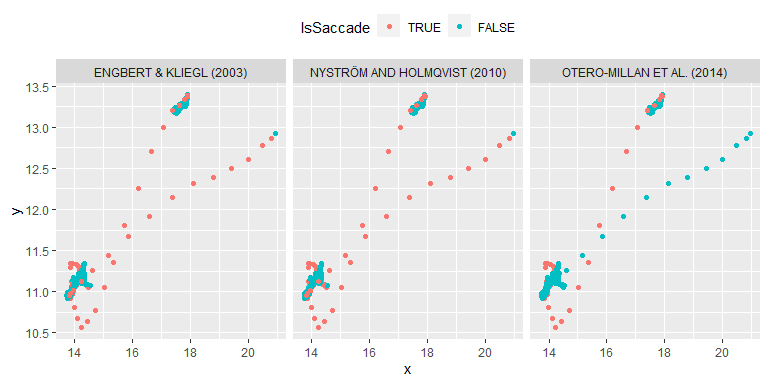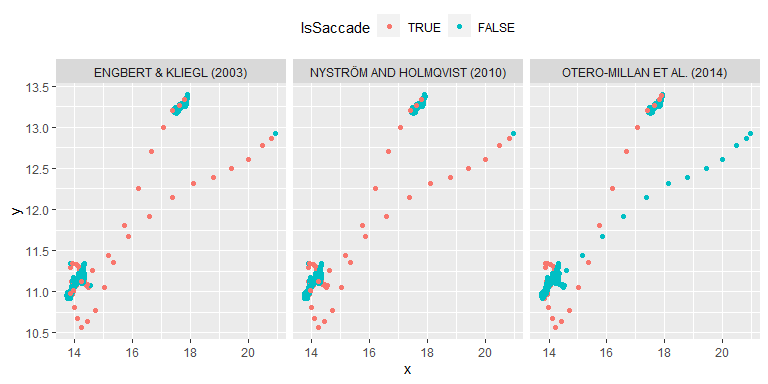library(ggplot2)
library(saccadr)

Function extract_saccades() has an option to return sample votes for each method via return_votes = TRUE parameter. Here is an example of using them for internally implemented methods.

data("single_trial")

methods_to_use <- list("Engbert & Kliegl (2003)" = method_ek,
"Otero-Millan et al. (2014)" = method_om,
"Nyström and Holmqvist (2010)" = method_nh)

votes <- extract_saccades(x = single_trial$x, y = single_trial$y,
sample_rate = 500,
methods = methods_to_use,

for(imethod in 1:length(methods_to_use)){
single_trial_with_votes[[imethod]]$Method <- toupper(names(methods_to_use)[imethod]) single_trial_with_votes[[imethod]]$IsSaccade <- votes[, imethod]
single_trial_with_votes[[imethod]]$IsSaccade <- factor(single_trial_with_votes[[imethod]]$IsSaccade == 1,
levels = c(TRUE, FALSE))
}

aes(x = x, y = y, color = IsSaccade)) +
geom_point() +
facet_grid(. ~ Method) +
theme(legend.position = "top")Same data but using Nyström and Holmqvist (2010) to compute velocity and acceleration

votes <- extract_saccades(x = single_trial$x, y = single_trial$y,
sample_rate = 500,
methods = methods_to_use,
velocity_function = diff_nh,

for(imethod in 1:length(methods_to_use)){
single_trial_with_votes[[imethod]]$Method <- toupper(names(methods_to_use)[imethod]) single_trial_with_votes[[imethod]]$IsSaccade <- votes[, imethod]
single_trial_with_votes[[imethod]]$IsSaccade <- factor(single_trial_with_votes[[imethod]]$IsSaccade == 1,
levels = c(TRUE, FALSE))
}

theme(legend.position = "top")